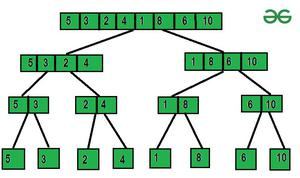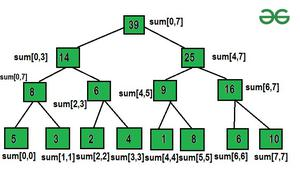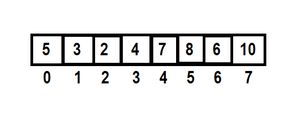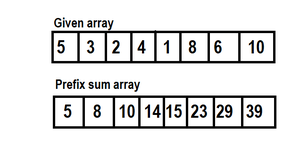Open in App
Not now

• Difficulty Level : Medium
• Last Updated : 20 Jan, 2023

First, let us understand why we need it prior to landing on the introduction so as to get why this concept was introduced. Suppose we are given an array and we need to find out the subarray

Purpose of Segment Trees:

A segment tree is a data structure that deals with a range of queries over an array. It has a divide and conquers approach. Used to solve range minimum and maximum & Sum Queries and Range Update Queries in O (log n) time complexity.

Construction of Segment Tree:

array[]: 5, 3, 2, 4, 1, 8, 6 10

we’ll use divide and conquerNow we’ll see how to segment the tree Construction is done.

Number of nodes will be = n + n/2 + n/4 + …… + 2+1

Geometric progression: common difference will be 2

let, number of terms be ‘x’, a=first term and r = common ratio.

(ar)^x-1 = n

a=1,r=2

(2)^x-1=n

log2(2)^x-1=log2n

x=1+log2n=number of levels

(2) – number of nodes = 1+2+4+….+n/2+n/4+n

1[(2)^1+logn – 1]/2-1 => (2n-1)Let us take an example of returning and updating the sum of the subarray a[i…..j] of size n.

Example:Query: output the sum from i=1 to i=5

Approach 1: Iterate from i = 1 to i = 5 and calculate the sum, update the element at i’th index, we’ll update a[i] = updated element. now, the time complexity of the query is O(n) and the update is O(1).

Approach 2: Prefix Sum approach

First, we’ll build the prefix sum array.Query: Output the sum from i to j

sum[i….j] = {pref[j] – pref[i-1]} (if i!=0)

pref[j] (if i=0)

Time Complexity : O(1)

Updating value: Put a[i] = updated value.

To update the prefix array we need to change all prefix[i].

Array:

i=4,

Prefix sum array:

Let’s take an example to understand this.

Example: Update the 4th index element to 13.

Original array: arr[]

Prefix sum: arr[] all elements get changed because the 4th index is updated so it will affect all elements after the 3rd index.

Time Complexity comparison table:

Requirement of log(n) time complexity: Many times, the number of queries and number of updates are of the order of 105-106, we will get TLE if we use Approach 1 or Approach 2.

Building a segment tree:

It is very simple to build a segment tree, we use the divide and conquer approach to build the segment tree.

pseudocode for implementation of Segment tree:

const int N = 1e5+2;
int a[N];
int tree[4*N];

void build(int node, int st, int en) {

if(st==en) {

tree[node] = a[st];
return;
}

int mid = (st+en)/2;
build(2*node, st, mid);
build(2*node+1, mid+1, en);

tree[node] = tree[2*node] + tree[2*node+1];
}

Applications of Segment trees:

Segment tree data structure can be used to solve various problems like:

•  Range Min, Max & Sum Queries, and Range Update Queries
•  segment tree with the max build, query, and update.
• segment tree with min build, query, and update.
• Computational geometry: Computational geometry is a mathematical field that includes the design, and analysis of efficient algorithms for solving geometric I/O problems. It is also used for pattern recognition and describes solid modeling algorithms.
• Geographic information systems: A Geographic Information System is a system that uses data that is attached to a unique location, and analyzes and generates geographically referenced information.
• Storing segments in an arbitrary manner.
• Used in competitive programming.
• Segment trees can be used to count the frequency of elements in a given range.
• : Segment trees can be used for image processing tasks.